## Breaking News

Google describes PageRank:

 “ PageRank reflects our view of the importance of web pages by considering more than 500 million variables and 2 billion terms. Pages that we believe are important pages receive a higher PageRank and are more likely to appear at the top of the search results. PageRank also considers the importance of each page that casts a vote, as votes from some pages are considered to have greater value, thus giving the linked page greater value. We have always taken a pragmatic approach to help improve search quality and create useful products, and our technology uses the collective intelligence of the web to determine a page's importance.”
A PageRank results from a mathematical algorithm based on the graph, the webgraph, created by all World Wide Web pages as nodes and hyperlinks as edges, taking into consideration authority hubs like Wikipedia (however, Wikipedia is actually a sink rather than a hub because it uses nofollow on external links). The rank value indicates an importance of a particular page. A hyperlink to a page counts as a vote of support. The PageRank of a page is defined recursively and depends on the number and PageRank metric of all pages that link to it ("incoming links"). A page that is linked to by many pages with high PageRank receives a high rank itself. If there are no links to a web page there is no support for that page.
Numerous academic papers concerning PageRank have been published since Page and Brin's original paper.
In practice, the PageRank concept has proven to be vulnerable to manipulation, and extensive research has been devoted to identifying falsely inflated PageRank and ways to ignore links from documents with falsely inflated PageRank.
Other link-based ranking algorithms for Web pages include the HITS algorithm invented by Jon Kleinberg (used by Teoma and now Ask.com), the IBM CLEVER project, and the TrustRank algorithm.

## History

PageRank was developed at Stanford University by Larry Page (hence the name Page-Rank) and Sergey Brin as part of a research project about a new kind of search engine. Sergey Brin had the idea that information on the web could be ordered in a hierarchy by "link popularity": a page is ranked higher as there are more links to it. It was co-authored by Rajeev Motwani and Terry Winograd. The first paper about the project, describing PageRank and the initial prototype of the Google search engine, was published in 1998: shortly after, Page and Brin founded Google Inc., the company behind the Google search engine. While just one of many factors that determine the ranking of Google search results, PageRank continues to provide the basis for all of Google's web search tools.
PageRank has been influenced by citation analysis, early developed by Eugene Garfield in the 1950s at the University of Pennsylvania, and by Hyper Search, developed by Massimo Marchiori at the University of Padua. In the same year PageRank was introduced (1998), Jon Kleinberg published his important work on HITS. Google's founders cite Garfield, Marchiori, and Kleinberg in their original paper.
A small search engine called "RankDex" from IDD Information Services designed by Robin Li was, since 1996, already exploring a similar strategy for site-scoring and page ranking. The technology in RankDex would be patented by 1999 and used later when Li founded Baidu in China. Li's work would be referenced by some of Larry Page's U.S. patents for his Google search methods.

## Algorithm

PageRank is a probability distribution used to represent the likelihood that a person randomly clicking on links will arrive at any particular page. PageRank can be calculated for collections of documents of any size. It is assumed in several research papers that the distribution is evenly divided among all documents in the collection at the beginning of the computational process. The PageRank computations require several passes, called "iterations", through the collection to adjust approximate PageRank values to more closely reflect the theoretical true value.
A probability is expressed as a numeric value between 0 and 1. A 0.5 probability is commonly expressed as a "50% chance" of something happening. Hence, a PageRank of 0.5 means there is a 50% chance that a person clicking on a random link will be directed to the document with the 0.5 PageRank.

### Simplified algorithm

Assume a small universe of four web pages: A, B, C and D. The initial approximation of PageRank would be evenly divided between these four documents. Hence, each document would begin with an estimated PageRank of 0.25.
In the original form of PageRank initial values were simply 1. This meant that the sum of all pages was the total number of pages on the web at that time. Later versions of PageRank (see the formulas below) would assume a probability distribution between 0 and 1. Here a simple probability distribution will be used—hence the initial value of 0.25.
If pages B, C, and D each only link to A, they would each confer 0.25 PageRank to A. All PageRank PR( ) in this simplistic system would thus gather to A because all links would be pointing to A.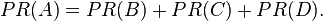$PR(A)= PR(B) + PR(C) + PR(D).\,$
This is 0.75.
Suppose that page B has a link to page C as well as to page A, while page D has links to all three pages. The value of the link-votes is divided among all the outbound links on a page. Thus, page B gives a vote worth 0.125 to page A and a vote worth 0.125 to page C. Only one third of D's PageRank is counted for A's PageRank (approximately 0.083).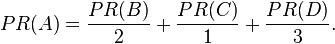$PR(A)= \frac{PR(B)}{2}+ \frac{PR(C)}{1}+ \frac{PR(D)}{3}.\,$
In other words, the PageRank conferred by an outbound link is equal to the document's own PageRank score divided by the normalized number of outbound links L( ) (it is assumed that links to specific URLs only count once per document).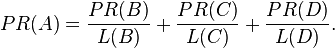$PR(A)= \frac{PR(B)}{L(B)}+ \frac{PR(C)}{L(C)}+ \frac{PR(D)}{L(D)}. \,$
In the general case, the PageRank value for any page u can be expressed as: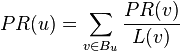$PR(u) = \sum_{v \in B_u} \frac{PR(v)}{L(v)}$,
i.e. the PageRank value for a page u is dependent on the PageRank values for each page v out of the set Bu (this set contains all pages linking to page u), divided by the number L(v) of links from page v.

### Damping factor

The PageRank theory holds that even an imaginary surfer who is randomly clicking on links will eventually stop clicking. The probability, at any step, that the person will continue is a damping factor d. Various studies have tested different damping factors, but it is generally assumed that the damping factor will be set around 0.85.
The damping factor is subtracted from 1 (and in some variations of the algorithm, the result is divided by the number of documents ( N ) in the collection) and this term is then added to the product of the damping factor and the sum of the incoming PageRank scores. That is,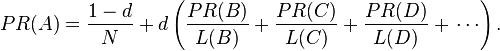$PR(A) = {1 - d \over N} + d \left( \frac{PR(B)}{L(B)}+ \frac{PR(C)}{L(C)}+ \frac{PR(D)}{L(D)}+\,\cdots \right).$
So any page's PageRank is derived in large part from the PageRanks of other pages. The damping factor adjusts the derived value downward. The original paper, however, gave the following formula, which has led to some confusion: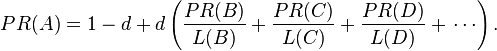$PR(A)= 1 - d + d \left( \frac{PR(B)}{L(B)}+ \frac{PR(C)}{L(C)}+ \frac{PR(D)}{L(D)}+\,\cdots \right).$
The difference between them is that the PageRank values in the first formula sum to one, while in the second formula each PageRank gets multiplied by N and the sum becomes N. A statement in Page and Brin's paper that "the sum of all PageRanks is one" and claims by other Google employees support the first variant of the formula above.
To be more specific, in the latter formula, the probability for the random surfer reaching a page is weighted by the total number of web pages. So, in this version PageRank is an expected value for the random surfer visiting a page, when he restarts this procedure as often as the web has pages. If the web had 100 pages and a page had a PageRank value of 2, the random surfer would reach that page in an average twice if he restarts 100 times. Basically, the two formulas do not differ fundamentally from each other. A PageRank that has been calculated by using the former formula has to be multiplied by the total number of web pages to get the according PageRank that would have been calculated by using the latter formula. Even Page and Brin mixed up the two formulas in their most popular paper "The Anatomy of a Large-Scale Hypertextual Web Search Engine", where they claim the latter formula to form a probability distribution over web pages with the sum of all pages' PageRanks being one.
Google recalculates PageRank scores each time it crawls the Web and rebuilds its index. As Google increases the number of documents in its collection, the initial approximation of PageRank decreases for all documents.
The formula uses a model of a random surfer who gets bored after several clicks and switches to a random page. The PageRank value of a page reflects the chance that the random surfer will land on that page by clicking on a link. It can be understood as a Markov chain in which the states are pages, and the transitions are all equally probable and are the links between pages.
If a page has no links to other pages, it becomes a sink and therefore terminates the random surfing process. If the random surfer arrives at a sink page, it picks another URL at random and continues surfing again.
When calculating PageRank, pages with no outbound links are assumed to link out to all other pages in the collection. Their PageRank scores are therefore divided evenly among all other pages. In other words, to be fair with pages that are not sinks, these random transitions are added to all nodes in the Web, with a residual probability of usually d = 0.85, estimated from the frequency that an average surfer uses his or her browser's bookmark feature.
So, the equation is as follows: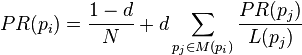$PR(p_i) = \frac{1-d}{N} + d \sum_{p_j \in M(p_i)} \frac{PR (p_j)}{L(p_j)}$
where p1,p2,...,pN are the pages under consideration, M(pi) is the set of pages that link to pi, L(pj) is the number of outbound links on page pj, and N is the total number of pages.
The PageRank values are the entries of the dominant eigenvector of the modified adjacency matrix. This makes PageRank a particularly elegant metric: the eigenvector is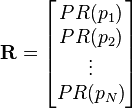$\mathbf{R} = \begin{bmatrix} PR(p_1) \\ PR(p_2) \\ \vdots \\ PR(p_N) \end{bmatrix}$
where R is the solution of the equation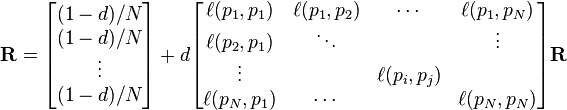$\mathbf{R} = \begin{bmatrix} {(1-d)/ N} \\ {(1-d) / N} \\ \vdots \\ {(1-d) / N} \end{bmatrix} + d \begin{bmatrix} \ell(p_1,p_1) & \ell(p_1,p_2) & \cdots & \ell(p_1,p_N) \\ \ell(p_2,p_1) & \ddots & & \vdots \\ \vdots & & \ell(p_i,p_j) & \\ \ell(p_N,p_1) & \cdots & & \ell(p_N,p_N) \end{bmatrix} \mathbf{R}$
where the adjacency function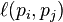$\ell(p_i,p_j)$ is 0 if page pj does not link to pi, and normalized such that, for each j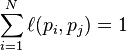$\sum_{i = 1}^N \ell(p_i,p_j) = 1$,
i.e. the elements of each column sum up to 1, so the matrix is a stochastic matrix (for more details see the computation section below). Thus this is a variant of the eigenvector centrality measure used commonly in network analysis.
Because of the large eigengap of the modified adjacency matrix above, the values of the PageRank eigenvector are fast to approximate (only a few iterations are needed).
As a result of Markov theory, it can be shown that the PageRank of a page is the probability of being at that page after lots of clicks. This happens to equal t − 1 where t is the expectation of the number of clicks (or random jumps) required to get from the page back to itself.
The main disadvantage is that it favors older pages, because a new page, even a very good one, will not have many links unless it is part of an existing site (a site being a densely connected set of pages, such as Wikipedia). The Google Directory (itself a derivative of the Open Directory Project) allows users to see results sorted by PageRank within categories. The Google Directory is the only service offered by Google where PageRank directly determines display order.[citation needed] In Google's other search services (such as its primary Web search) PageRank is used to weight the relevance scores of pages shown in search results.
Several strategies have been proposed to accelerate the computation of PageRank.
Various strategies to manipulate PageRank have been employed in concerted efforts to improve search results rankings and monetize advertising links. These strategies have severely impacted the reliability of the PageRank concept, which seeks to determine which documents are actually highly valued by the Web community.
Google is known to penalize link farms and other schemes designed to artificially inflate PageRank. In December 2007 Google started actively penalizing sites selling paid text links. How Google identifies link farms and other PageRank manipulation tools are among Google's trade secrets.

### Computation

To summarize, PageRank can be either computed iteratively or algebraically. The iterative method can be viewed differently as the power iteration method, or power method. The basic mathematical operations performed in the iterative method and the power method are identical.

#### Iterative

In the former case, at t = 0, an initial probability distribution is assumed, usually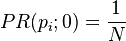$PR(p_i; 0) = \frac{1}{N}$.
At each time step, the computation, as detailed above, yields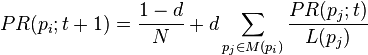$PR(p_i;t+1) = \frac{1-d}{N} + d \sum_{p_j \in M(p_i)} \frac{PR (p_j; t)}{L(p_j)}$,
or in matrix notation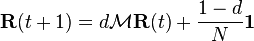$\mathbf{R}(t+1) = d \mathcal{M}\mathbf{R}(t) + \frac{1-d}{N} \mathbf{1}$,       (*)
where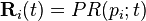$\mathbf{R}_i(t)=PR(p_i; t)$ and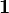$\mathbf{1}$ is the column vector of length N containing only ones.
The matrix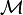$\mathcal{M}$ is defined as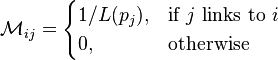$\mathcal{M}_{ij} = \begin{cases} 1 /L(p_j) , & \mbox{if }j\mbox{ links to }i\ \\ 0, & \mbox{otherwise} \end{cases}$
i.e.,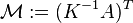$\mathcal{M} := (K^{-1} A)^T$,
where A denotes the adjacency matrix of the graph and K is the diagonal matrix with the outdegrees in the diagonal.
The computation ends when for some small ε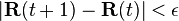$|\mathbf{R}(t+1) - \mathbf{R}(t)| < \epsilon$,
i.e., when convergence is assumed.

#### Algebraic

In the latter case, for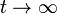$t \to \infty$ (i.e., in the steady state), the above equation (*) reads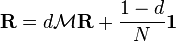$\mathbf{R} = d \mathcal{M}\mathbf{R} + \frac{1-d}{N} \mathbf{1}$.       (**)
The solution is given by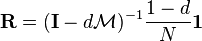$\mathbf{R} = (\mathbf{I}-d \mathcal{M})^{-1} \frac{1-d}{N} \mathbf{1}$,
with the identity matrix$\mathbf{I}$.
The solution exists and is unique for 0 < d < 1. This can be seen by noting that$\mathcal{M}$ is by construction a stochastic matrix and hence has an eigenvalue equal to one because of the Perron–Frobenius theorem.

#### Power Method

If the matrix$\mathcal{M}$ is a transition probability, i.e., column-stochastic with no columns consisting of just zeros and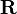$\mathbf{R}$ is a probability distribution (i.e.,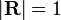$|\mathbf{R}|=1$,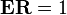$\mathbf{E}\mathbf{R}=1$ where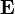$\mathbf{E}$ is matrix of all ones), Eq. (**) is equivalent to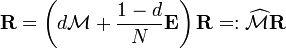$\mathbf{R} = \left( d \mathcal{M} + \frac{1-d}{N} \mathbf{E} \right)\mathbf{R} =: \widehat \mathcal{M} \mathbf{R}$.       (***)
Hence PageRank$\mathbf{R}$ is the principal eigenvector of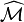$\widehat\mathcal{M}$. A fast and easy way to compute this is using the power method: starting with an arbitrary vector x(0), the operator$\widehat\mathcal{M}$ is applied in succession, i.e.,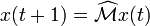$x(t+1) = \widehat\mathcal{M} x(t)$,
until
| x(t + 1) − x(t) | < ε.
Note that in Eq. (***) the matrix on the right-hand side in the parenthesis can be interpreted as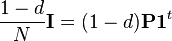$\frac{1-d}{N} \mathbf{I} = (1-d)\mathbf{P} \mathbf{1}^t$,
where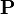$\mathbf{P}$ is an initial probability distribution. In the current case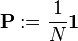$\mathbf{P} := \frac{1}{N} \mathbf{1}$.
Finally, if$\mathcal{M}$ has columns with only zero values, they should be replaced with the initial probability vector$\mathbf{P}$. In other words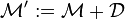$\mathcal{M}^\prime := \mathcal{M} + \mathcal{D}$,
where the matrix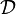$\mathcal{D}$ is defined as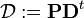$\mathcal{D} := \mathbf{P} \mathbf{D}^t$,
with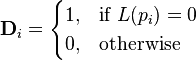$\mathbf{D}_i = \begin{cases} 1, & \mbox{if }L(p_i)=0\ \\ 0, & \mbox{otherwise} \end{cases}$
In this case, the above two computations using$\mathcal{M}$ only give the same PageRank if their results are normalized: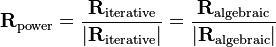$\mathbf{R}_{\textrm{power}} = \frac{\mathbf{R}_{\textrm{iterative}}}{|\mathbf{R}_{\textrm{iterative}}|} = \frac{\mathbf{R}_{\textrm{algebraic}}}{|\mathbf{R}_{\textrm{algebraic}}|}$.

#### Efficiency

Depending on the framework used to perform the computation, the exact implementation of the methods, and the required accuracy of the result, the computation time of the these methods can vary greatly.